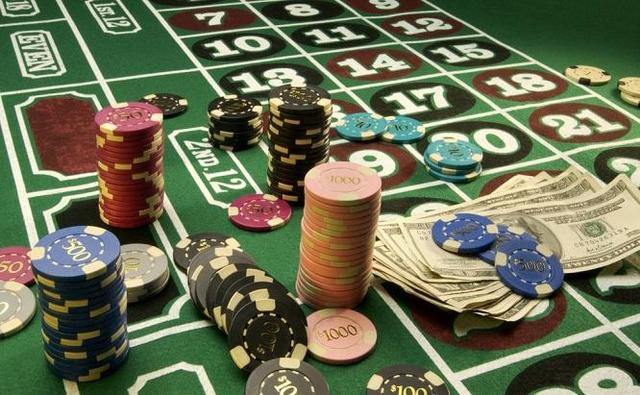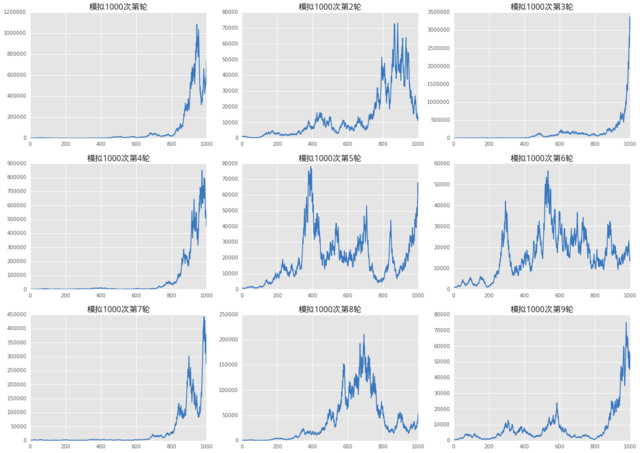首页   注册   登录
V2EX = way to explore
V2EX 是一个关于分享和探索的地方

Learn Python the Hard Way
Python 学习手册Python CookbookPython 基础教程Python Sites
PyPI - Python Package Index
http://www.simple-is-better.com/
http://diveintopython.org/toc/index.html
Pocoo

PyPy
Celery
Jinja2
gevent
pyenv
virtualenv
Stackless Python
Beautiful Soup

Green Unicorn
Sentry
Shovel
Pyflakes
pytest
Python 编程
pep8 Checker
Styles
PEP 8
Code Style from The Hitchhiker's Guide
V2EX  ›  Python

# 基于凯利公式的 21 点 仓位管理玩法

datayes2015 · 2016-09-07 15:55:33 +08:00 · 2334 次点击rw = 1

rl = -1

p = 0.55

f = (p/abs(rl) -(1-p)/abs(rw))*100

print('则最佳理论值 f 为: &# 39; + '%0.2f&# 39;%(f) + '%&# 39;)

import random

import numpy as np

import pandas as pd

import matplotlib as mpl

import matplotlib.pyplot as plt

mpl.style.use('ggplot&# 39;)

import seaborn as sns

from CAL.PyCAL import font

import random

def runOneTurnGame(rw, rl, p):

assets = 

f=p/abs(rl)-(1-p)/abs(rw)

for i in range(1000):

lastAsset = assets[-1]

isWin = True if random.random() < p else False

if isWin:

currentAsset = lastAsset*(1-f) + lastAsset*f*(1+rw)

else:

currentAsset = lastAsset*(1-f) + lastAsset*f*(1+rl)

assets.append(currentAsset)

return assets